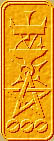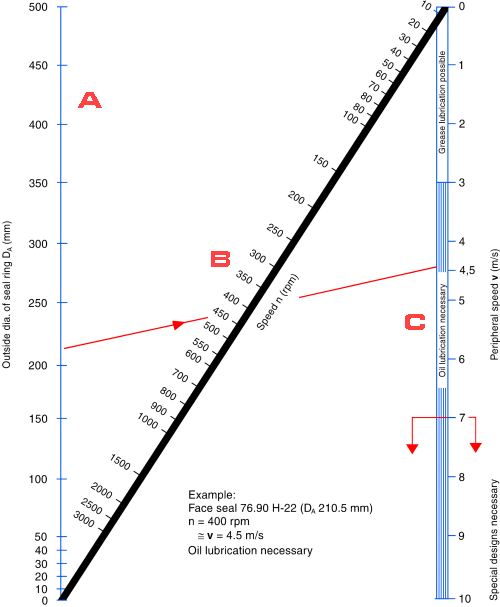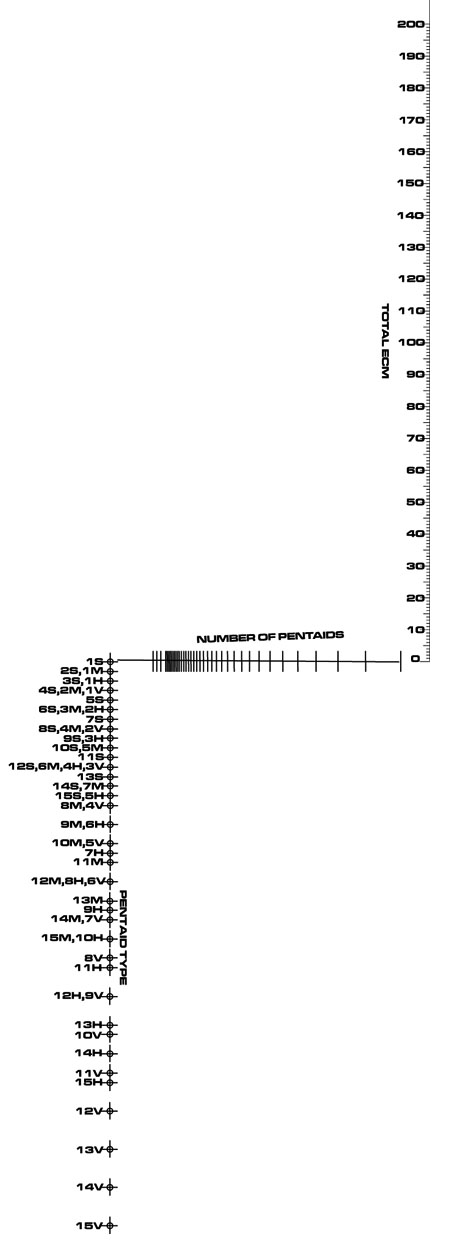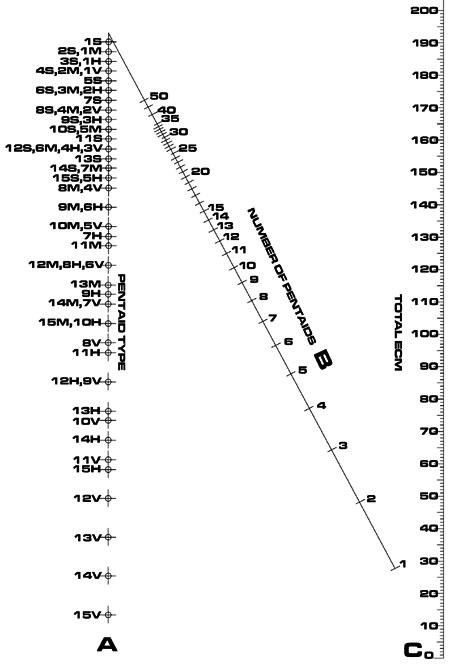Nomography Main > N Nomogramsρ=Σ+Ψ
``` <!-- RegisterNomoPage("ntype.html") PrintNomoHeader("Reference", "Antique Instruments"); PrintNomoPageNum() //--> ```

# "N" or "Z" type Nomograms

These nomograms are for multiplying or dividing. As a rule of thumb these are more inaccurate than a logarithmic parallel scale nomogram, which also can multiply and divide. Their only saving grace is that it is possible to draw one with no calculation at all, using only ruler and pencil.If the above nomogram had equal spacing on the scales, it would calculate B = C / A, or C = A * B. Note how the A and C scales have their zero points where they are cut by the diagonal B scale. Note that the zero point on the diagonal B scale overlaps the zero point on the C scale. This lets you know that the equation is B = C / A, not B = A / C.

The scales cannot be logarithmic, though you can get something close by spacing the tick marks raised to a power.

A peek at the cheat sheet for N type nomograms will reveal the following constructional determinant:

 0 μ1 . f(u) 1 {δ3 . μ1 { f(v) / { f(v)+1} } } / {{μ1 - μ3}{ f(v) / { f(v)+1} } + μ3} 0 1 δ3 -μ3 . f(w) 1

Translated into English:

 X coordequation Y coordequation U scale 0 μ1 * f(u) V scale {δ3 * μ1 { f(v) / { f(v)+1} } } / {{μ1 - μ3}{ f(v) / { f(v)+1} } + μ3} 0 W scale δ3 -μ3 * f(w)

Unlike the parallel scale chart, there are no limitations on the functional moduli

Plotted as specified, the nomogram becomes a most inconvenient shape:Not only does this waste paper, it forces the scales to be printed so tiny that they yield inaccurate results. However, there is trick called Oblique Axes which can squeeze the nomogram into a more efficient shape:(Note: the two nomograms above are actually mirror images of what you get when you plot them. I had inverted them in preparation of making a compound nomogram and I didn't feel like re-doing them for the illustration.)

For this nomogram, we have to shift the scales upward. Examine the U scale (it's the one where X coord is always zero and extends above the y axis). Make a note of the largest value for Y coord in the U scale. Call this "Oblique".

The equation for the V scale Y coord becomes: 0 + { X-coord * Oblique }

The equation for the W scale Y coord becomes: { -μ3 * f(w) } + { X-coord * Oblique }

(Technically this applies to the U scale as well, but since the X coord is always zero, so is { X-coord * Oblique }. Just ignore it.)

Applying the oblique factor will make all three scales of the N nomogram line up at the top.

How do you draw an N nomogram without calculation? Draw the two side scales, and the diagonal middle line. Then use a straight-edge across various values for the side scales, and draw a tick mark where it crosses the diagonal line. Label the tick mark with the proper value. Repeat until all the tick marks are present.